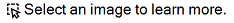Calculations

Relevant for: GUI actions, scripted GUI components, and function librariesYou can write statements that perform simple calculations using mathematical operators. For example, you can use a multiplication operator to multiply the values displayed in two text boxes in your Web site. VBScript supports the following mathematical operators:

Operator

Description

+

subtraction

negation (a negative number)

*

multiplication

/

division

^

exponent

In the following example, the multiplication operator is used to calculate the total price of three speakers:

'Retrieves the price of a speaker using the GetROProperty method